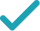Type a math problemSolve for xSteps Using the Quadratic Formula
Steps for Completing the Square
All equations of the form can be solved using the quadratic formula: . The quadratic formula gives two solutions, one when is addition and one when it is subtraction.
Subtract from both sides of the equation.
Subtracting from itself leaves .
Subtract from .
This equation is in standard form: . Substitute for , for , and for in the quadratic formula, .
Square .
Multiply times .
Multiply times .
Take the square root of .
Multiply times .
Now solve the equation when is plus. Add to .
Divide by .
Now solve the equation when is minus. Subtract from .
Divide by .
The equation is now solved.
Solve for ySteps for Solving Linear Equation
Subtract from both sides.
Subtract from both sides.
Subtract from to get .
Divide both sides by .
Dividing by undoes the multiplication by .
Divide by .
GraphGiving is as easy as 1, 2, 3
Get 1,000 points to donate to a school of your choice when you join Give With Bing
24x^{2}+16yx+8=84
All equations of the form ax^{2}+bx+c=0 can be solved using the quadratic formula: \frac{-b±\sqrt{b^{2}-4ac}}{2a}. The quadratic formula gives two solutions, one when ± is addition and one when it is subtraction.
24x^{2}+16yx+8-84=84-84
Subtract 84 from both sides of the equation.
24x^{2}+16yx+8-84=0
Subtracting 84 from itself leaves 0.
24x^{2}+16yx-76=0
Subtract 84 from 8.
x=\frac{-16y±\sqrt{\left(16y\right)^{2}-4\times 24\left(-76\right)}}{2\times 24}
This equation is in standard form: ax^{2}+bx+c=0. Substitute 24 for a, 16y for b, and -76 for c in the quadratic formula, \frac{-b±\sqrt{b^{2}-4ac}}{2a}.
x=\frac{-16y±\sqrt{256y^{2}-4\times 24\left(-76\right)}}{2\times 24}
Square 16y.
x=\frac{-16y±\sqrt{256y^{2}-96\left(-76\right)}}{2\times 24}
Multiply -4 times 24.
x=\frac{-16y±\sqrt{256y^{2}+7296}}{2\times 24}
Multiply -96 times -76.
x=\frac{-16y±8\sqrt{4y^{2}+114}}{2\times 24}
Take the square root of 256y^{2}+7296.
x=\frac{-16y±8\sqrt{4y^{2}+114}}{48}
Multiply 2 times 24.
x=\frac{8\sqrt{4y^{2}+114}-16y}{48}
Now solve the equation x=\frac{-16y±8\sqrt{4y^{2}+114}}{48} when ± is plus. Add -16y to 8\sqrt{4y^{2}+114}.
x=\frac{\sqrt{4y^{2}+114}}{6}-\frac{y}{3}
Divide -16y+8\sqrt{4y^{2}+114} by 48.
x=\frac{-8\sqrt{4y^{2}+114}-16y}{48}
Now solve the equation x=\frac{-16y±8\sqrt{4y^{2}+114}}{48} when ± is minus. Subtract 8\sqrt{4y^{2}+114} from -16y.
x=-\frac{\sqrt{4y^{2}+114}}{6}-\frac{y}{3}
Divide -16y-8\sqrt{4y^{2}+114} by 48.
x=\frac{\sqrt{4y^{2}+114}}{6}-\frac{y}{3} x=-\frac{\sqrt{4y^{2}+114}}{6}-\frac{y}{3}
The equation is now solved.
24x^{2}+16yx+8=84
Quadratic equations such as this one can be solved by completing the square. In order to complete the square, the equation must first be in the form x^{2}+bx=c.
24x^{2}+16yx+8-8=84-8
Subtract 8 from both sides of the equation.
24x^{2}+16yx=84-8
Subtracting 8 from itself leaves 0.
24x^{2}+16yx=76
Subtract 8 from 84.
\frac{24x^{2}+16yx}{24}=\frac{76}{24}
Divide both sides by 24.
x^{2}+\frac{16y}{24}x=\frac{76}{24}
Dividing by 24 undoes the multiplication by 24.
x^{2}+\frac{2y}{3}x=\frac{76}{24}
Divide 16y by 24.
x^{2}+\frac{2y}{3}x=\frac{19}{6}
Reduce the fraction \frac{76}{24}\approx 3.166666667 to lowest terms by extracting and canceling out 4.
x^{2}+\frac{2y}{3}x+\left(\frac{y}{3}\right)^{2}=\frac{19}{6}+\left(\frac{y}{3}\right)^{2}
Divide \frac{2y}{3}, the coefficient of the x term, by 2 to get \frac{y}{3}. Then add the square of \frac{y}{3} to both sides of the equation. This step makes the left hand side of the equation a perfect square.
x^{2}+\frac{2y}{3}x+\frac{y^{2}}{9}=\frac{19}{6}+\frac{y^{2}}{9}
Square \frac{y}{3}.
x^{2}+\frac{2y}{3}x+\frac{y^{2}}{9}=\frac{y^{2}}{9}+\frac{19}{6}
\left(x+\frac{y}{3}\right)^{2}=\frac{y^{2}}{9}+\frac{19}{6}
Factor x^{2}+\frac{2y}{3}x+\frac{y^{2}}{9}. In general, when x^{2}+bx+c is a perfect square, it can always be factored as \left(x+\frac{b}{2}\right)^{2}.
\sqrt{\left(x+\frac{y}{3}\right)^{2}}=\sqrt{\frac{y^{2}}{9}+\frac{19}{6}}
Take the square root of both sides of the equation.
x+\frac{y}{3}=\frac{\sqrt{4y^{2}+114}}{6} x+\frac{y}{3}=-\frac{\sqrt{4y^{2}+114}}{6}
Simplify.
x=\frac{\sqrt{4y^{2}+114}}{6}-\frac{y}{3} x=-\frac{\sqrt{4y^{2}+114}}{6}-\frac{y}{3}
Subtract \frac{y}{3} from both sides of the equation.
16xy+8=84-24x^{2}
Subtract 24x^{2} from both sides.
16xy=84-24x^{2}-8
Subtract 8 from both sides.
16xy=76-24x^{2}
Subtract 8 from 84 to get 76.
\frac{16xy}{16x}=\frac{76-24x^{2}}{16x}
Divide both sides by 16x.
y=\frac{76-24x^{2}}{16x}
Dividing by 16x undoes the multiplication by 16x.
y=-\frac{3x}{2}+\frac{19}{4x}
Divide 76-24x^{2} by 16x.